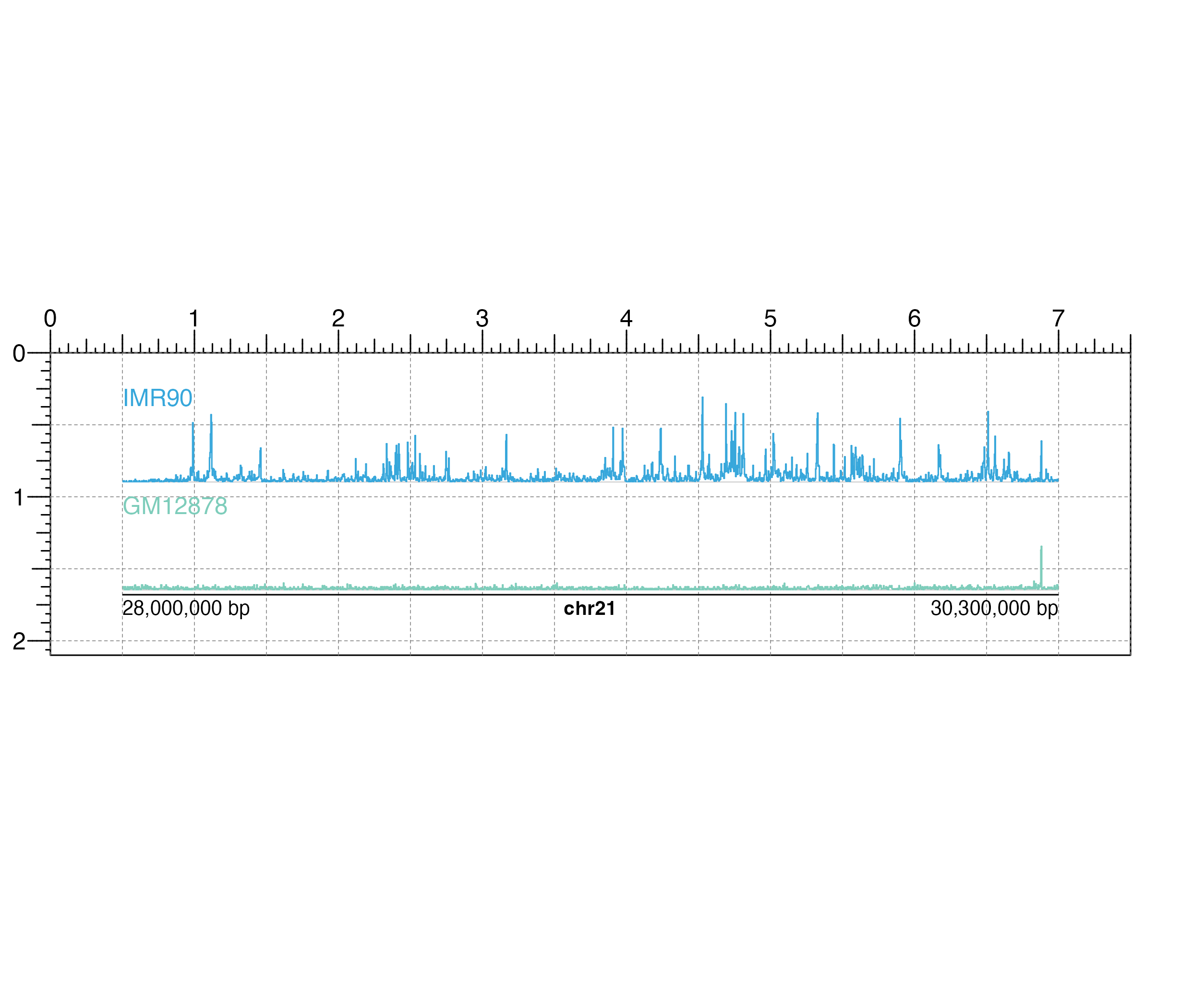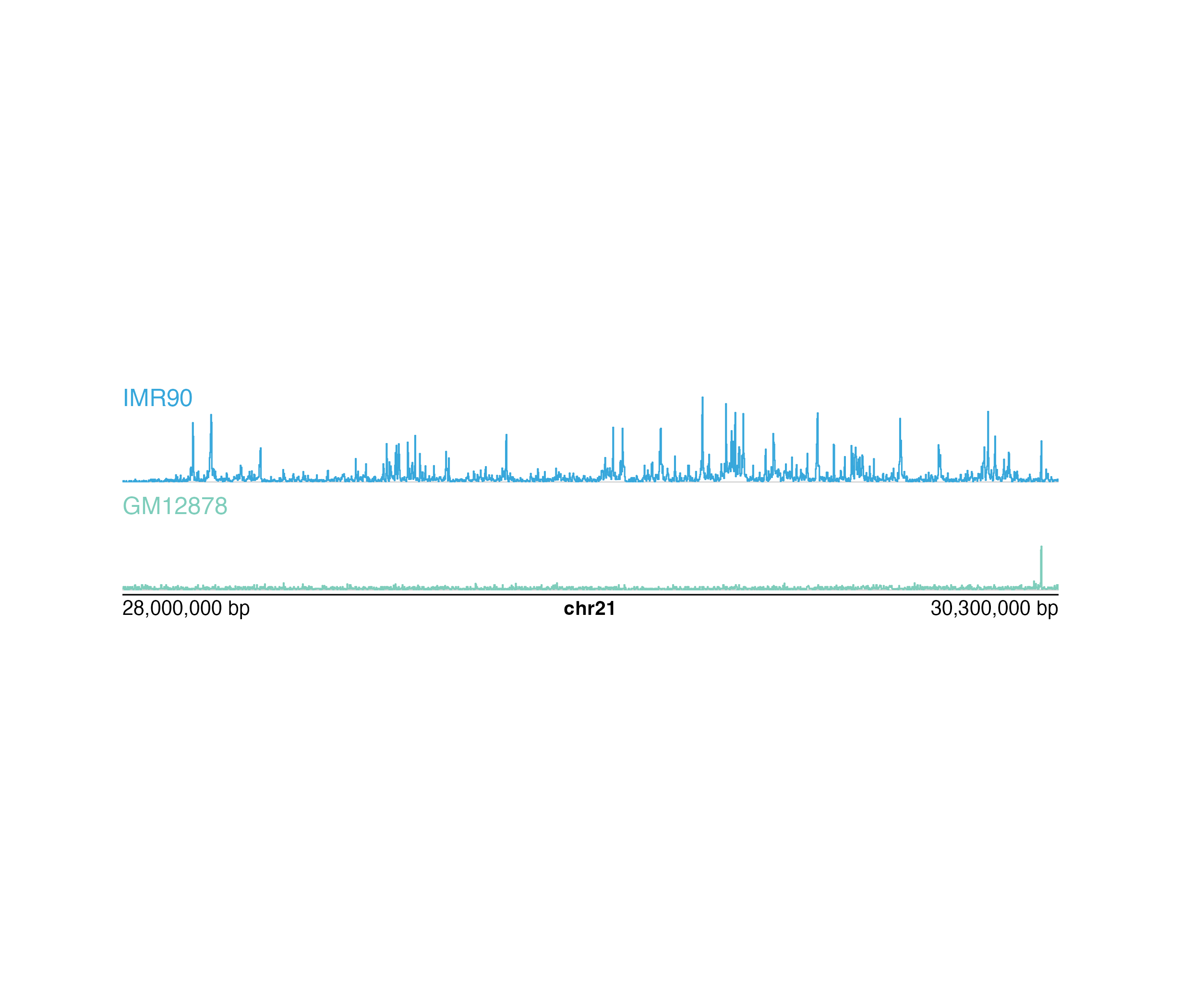Plot any kind of signal track data for a single chromosome

plotSignal(
data,
binSize = NA,
binCap = TRUE,
negData = FALSE,
chrom,
chromstart = NULL,
chromend = NULL,
assembly = "hg38",
linecolor = "#37a7db",
fill = NA,
ymax = 1,
range = NULL,
scale = FALSE,
bg = NA,
baseline = TRUE,
baseline.color = "grey",
baseline.lwd = 1,
orientation = "h",
x = NULL,
y = NULL,
width = NULL,
height = NULL,
just = c("left", "top"),
default.units = "inches",
draw = TRUE,
params = NULL,
...
)

## Arguments

data

Data to be plotted as a character value specifying a bigwig file path, a dataframe in BED format, or a GRanges object with metadata column score. Either one data argument or a list of two can be provided, where the second data will be plotted below the x-axis.

binSize

A numeric specifying the length of each data bin in basepairs. Default value is binSize = NA.

binCap

A logical value indicating whether the function will limit the number of data bins to 8,000. Default value is binCap = TRUE.

negData

A logical value indicating whether the data has both positive and negative scores and the y-axis should be split. Default value is negData = FALSE.

chrom

Chromosome of region to be plotted, as a string.

chromstart

Integer start position on chromosome to be plotted.

chromend

Integer end position on chromosome to be plotted.

assembly

Default genome assembly as a string or a assembly object. Default value is assembly = "hg38".

linecolor

A character value or vector of length 2 specifying the line color(s) outlining the signal track(s). Default value is linecolor = "#37a7db".

fill

A character value or vector of length 2 specifying the fill color(s) of the signal track(s). Default value is fill = NA.

ymax

A numeric specifying the fraction of the max y-value to set as the height of the plot. Default value is ymax = 1.

range

A numeric vector of length 2 specifying the y-range of data to plot (c(min, max)).

scale

A logical value indicating whether to include a data scale label in the top left corner of the plot. Default value is scale = FALSE.

bg

Character value indicating background color. Default value is bg = NA.

baseline

Logical value indicating whether to include a baseline along the x-axis. Default value is baseline = TRUE.

baseline.color

Baseline color. Default value is baseline.color = "grey".

baseline.lwd

Baseline line width. Default value is baseline.lwd = 1.

orientation

A string specifying signal track orientation. Default value is orientation = "h". Options are:

• "v": Vertical signal track orientation.

• "h": Horizontal signal track orientation.

x

A numeric or unit object specifying signal plot x-location.

y

A numeric, unit object, or character containing a "b" combined with a numeric value specifying signal plot y-location. The character value will place the signal plot y relative to the bottom of the most recently plotted plot according to the units of the plotgardener page.

width

A numeric or unit object specifying signal plot width.

height

A numeric or unit object specifying signal plot height.

just

Justification of signal plot relative to its (x, y) location. If there are two values, the first value specifies horizontal justification and the second value specifies vertical justification. Possible string values are: "left", "right", "centre", "center", "bottom", and "top". Default value is just = c("left", "top").

default.units

A string indicating the default units to use if x, y, width, or height are only given as numerics. Default value is default.units = "inches".

draw

A logical value indicating whether graphics output should be produced. Default value draw = TRUE.

params

An optional pgParams object containing relevant function parameters.

...

Additional grid graphical parameters. See gpar.

## Value

Returns a signal object containing relevant genomic region, placement, and grob information.

## Details

#A signal track can be placed on a plotgardener coordinate page by providing plot placement parameters:

plotSignal(data, chrom,
chromstart = NULL, chromend = NULL,
x, y, width, height, just = c("left", "top"),
default.units = "inches")

This function can also be used to quickly plot an unannotated signal track by ignoring plot placement parameters:

plotSignal(data, chrom,
chromstart = NULL, chromend = NULL)

## Examples

## Load signal data
library(plotgardenerData)
data("IMR90_ChIP_H3K27ac_signal")
data("GM12878_ChIP_H3K27ac_signal")

## Create a page
pageCreate(width = 7.5, height = 2.1, default.units = "inches")

## Define region
region <- pgParams(
chrom = "chr21",
chromstart = 28000000, chromend = 30300000,
assembly = "hg19",
range = c(0, 45)
)

## Plot and place signal plots
signal1 <- plotSignal(
data = IMR90_ChIP_H3K27ac_signal, params = region,
x = 0.5, y = 0.25, width = 6.5, height = 0.65,
just = c("left", "top"), default.units = "inches"
)
#> signal[signal1]

signal2 <- plotSignal(
data = GM12878_ChIP_H3K27ac_signal, params = region,
linecolor = "#7ecdbb",
x = 0.5, y = 1, width = 6.5, height = 0.65,
just = c("left", "top"), default.units = "inches"
)
#> signal[signal2]

## Plot genome label
plotGenomeLabel(
chrom = "chr21",
chromstart = 28000000, chromend = 30300000,
assembly = "hg19",
x = 0.5, y = 1.68, length = 6.5,
default.units = "inches"
)
#> genomeLabel[genomeLabel1]

plotText(
label = "IMR90", fonsize = 10, fontcolor = "#37a7db",
x = 0.5, y = 0.25, just = c("left", "top"),
default.units = "inches"
)
#> text[text1]
plotText(
label = "GM12878", fonsize = 10, fontcolor = "#7ecdbb",
x = 0.5, y = 1, just = c("left", "top"),
default.units = "inches"
)
#> text[text2]## Hide page guides
pageGuideHide()